# Phosphate

A phosphate, in inorganic chemistry, is a salt of phosphoric acid. In organic chemistry, a phosphate, or organophosphate, is an ester of phosphoric acid. Phosphates are important in biochemistry and biogeochemistry.

## Occurrence

Phosphates are the naturally occurring form of the element phosphorus, found in many phosphate minerals. Elemental phosphorus and phosphides are not found (rare phosphide minerals may be found in meteorites). In mineralogy and geology, phosphate refers to a rock or ore containing phosphate ions.

The largest rock phosphate deposits in North America lie in the Bone Valley region of central Florida, United States, the Soda Springs region of Idaho, and the coast of North Carolina. Smaller deposits are located in Montana, Tennessee, Georgia, and South Carolina near Charleston along Ashley Phosphate road. The small island nation of Nauru and its neighbor Banaba Island, which used to have massive phosphate deposits of the best quality, have been mined excessively. Rock phosphate can also be found on Navassa Island. Morocco, Tunisia, Israel, Togo, and Jordan have large phosphate mining industries as well.

In biological systems, phosphorus is found as a free phosphate ion in solution and is called inorganic phosphate, to distinguish it from phosphates bound in various phosphate esters. Inorganic phosphate is generally denoted Pi and can be created by the hydrolysis of pyrophosphate, which is denoted PPi:

P2O74− + H2O → 2HPO42−

However, phosphates are most commonly found in the form of adenosine phosphates, (AMP, ADP and ATP) and in DNA and RNA and can be released by the hydrolysis of ATP or ADP. Similar reactions exist for the other nucleoside diphosphates and triphosphates. Phosphoanhydride bonds in ADP and ATP, or other nucleoside diphosphates and triphosphates, contain high amounts of energy which give them their vital role in all living organisms. They are generally referred to as high energy phosphate, as are the phosphagens in muscle tissue. Compounds such as substituted phosphines, have uses in organic chemistry but do not seem to have any natural counterparts.

In ecological terms, because of its important role in biological systems, phosphate is a highly sought after resource. Consequently, it is often a limiting reagent in environments, and its availability may govern the rate of growth of organisms. Addition of high levels of phosphate to environments and to micro-environments in which it is typically rare can have significant ecological consequences; for example, booms in the populations of some organisms at the expense of others, and the collapse of populations deprived of resources such as oxygen (see eutrophication). In the context of pollution, phosphates are a principal component of total dissolved solids, a major indicator of water quality.

## Chemical propertiesThis is the structural formula of the phosphoric acid functional group as found in a weakly acidic aqueous solution. In more basic aqueous solutions, the group will donate the two hydrogen atoms and ionize as a phosphate group with a negative charge of 2. 

The phosphate ion is a polyatomic ion with the empirical formula PO43− and a molar mass of 94.973 g/mol; it consists of one central phosphorus atom surrounded by four identical oxygen atoms in a tetrahedral arrangement. The phosphate ion carries a negative three formal charge and is the conjugate base of the hydrogenphosphate ion, HPO42−, which is the conjugate base of H2PO4, the dihydrogen phosphate ion, which in turn is the conjugate base of H3PO4, phosphoric acid. It is a hypervalent molecule (the phosphorus atom has 10 electrons in its valence shell). Phosphate is also an organophosphorus compound with the formula OP(OR)3

A phosphate salt forms when a positively charged ion attaches to the negatively charged oxygen atoms of the ion, forming an ionic compound. Many phosphates are insoluble in water at standard temperature and pressure, except for the alkali metal salts.

In a dilute aqueous solution, phosphate exists in four forms. In strongly basic conditions, the phosphate ion (PO43−) predominates, while in weakly basic conditions, the hydrogen phosphate ion (HPO42−) is prevalent. In weakly acid conditions, the dihydrogen phosphate ion (H2PO4) is most common. In strongly acid conditions, aqueous phosphoric acid (H3PO4) is the main form.

Phosphate can form many polymeric ions, diphosphate (also pyrophosphate), P2O74−, triphosphate, P3O105−, and so forth. The various metaphosphate ions have an empirical formula of PO3 and are found in many compounds.

Phosphate deposits can contain significant amounts of naturally occurring uranium. Subsequent uptake of such soil amendments can lead to crops containing uranium concentrations.

## Uses

Phosphates were once commonly used in laundry detergent in the form trisodium phosphate (TSP), but because of algae boom-bust cycles tied to emission of phosphates into watersheds, phosphate detergent sale or usage is restricted in some areas.

In agriculture phosphate refers to one of the three primary plant nutrients, and it is a component of fertilizers. Rock phosphate is quarried from phosphate beds in sedimentary rocks. In former times, it was simply crushed and used as is, but the crude form is now used only in organic farming. Normally, it is chemically treated to make superphosphate, triple superphosphate, or ammonium phosphates, which have higher concentration of phosphate and are also more soluble, therefore more quickly usable by plants.

Fertilizer grades normally have three numbers; the first is the available nitrogen, the second is the available phosphate (expressed on a P2O5 basis), and the third is the available potash (expressed on a K2O basis). Thus, a 10-10-10 fertilizer would contain ten percent of each, with the remainder being filler.

Surface runoff of phosphates from excessively fertilized farmland can be a cause of phosphate pollution leading to eutrophication (nutrient enrichment), algal bloom and consequent oxygen deficit. This can lead to anoxia for fish and other aquatic organisms in the same manner as phosphate-based detergents.

Phosphate compounds are occasionally added to the public drinking water supply to counter plumbosolvency.

## Phosphate species at different pH values

The dissociation of phosphoric acid takes place in stages, generating various phosphate species. As the pH of the solution is changed, different phosphate species become dominant in the solution. Consider the following three equilibrium reactions:

H3PO4 ⇌ H+ + H2PO4
H2PO4 ⇌ H+ + HPO42−
HPO42− ⇌ H+ + PO43−

the corresponding constants at 25°C (in mol/L) are (see phosphoric acid):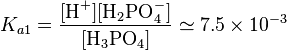$K_{a1}=\frac{[\mbox{H}^+][\mbox{H}_2\mbox{PO}_4^-]}{[\mbox{H}_3\mbox{PO}_4]}\simeq 7.5\times10^{-3}$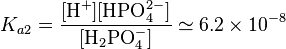$K_{a2}=\frac{[\mbox{H}^+][\mbox{HPO}_4^{2-}]}{[\mbox{H}_2\mbox{PO}_4^-]}\simeq 6.2\times10^{-8}$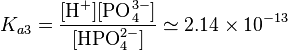$K_{a3}=\frac{[\mbox{H}^+][\mbox{PO}_4^{3-}]}{[\mbox{HPO}_4^{2-}]}\simeq 2.14\times10^{-13}$

In a strongly basic solution (pH=13):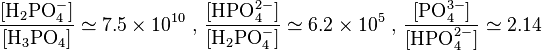$\frac{[\mbox{H}_2\mbox{PO}_4^-]}{[\mbox{H}_3\mbox{PO}_4]}\simeq 7.5\times10^{10} \mbox{ , }\frac{[\mbox{HPO}_4^{2-}]}{[\mbox{H}_2\mbox{PO}_4^-]}\simeq 6.2\times10^5 \mbox{ , } \frac{[\mbox{PO}_4^{3-}]}{[\mbox{HPO}_4^{2-}]}\simeq 2.14$

These ratios show that only PO43− and HPO42− are in significant amounts at high pH.

In a solution at neutral pH (pH=7.0, such as in the cytosol):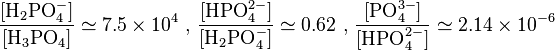$\frac{[\mbox{H}_2\mbox{PO}_4^-]}{[\mbox{H}_3\mbox{PO}_4]}\simeq 7.5\times10^4 \mbox{ , }\frac{[\mbox{HPO}_4^{2-}]}{[\mbox{H}_2\mbox{PO}_4^-]}\simeq 0.62 \mbox{ , } \frac{[\mbox{PO}_4^{3-}]}{[\mbox{HPO}_4^{2-}]}\simeq 2.14\times10^{-6}$

The above ratios indicate that only H2PO4 and HPO42− ions are in significant amounts (62% H2PO4, 38% HPO42−) at neutral pH. Note that in the extracellular fluid (pH=7.4), this proportion is inverted: 61% HPO42−, 39% H2PO4.

In a strongly acidic solution (pH=1):$\frac{[\mbox{H}_2\mbox{PO}_4^-]}{[\mbox{H}_3\mbox{PO}_4]}\simeq 0.075 \mbox{ , }\frac{[\mbox{HPO}_4^{2-}]}{[\mbox{H}_2\mbox{PO}_4^-]}\simeq 6.2\times10^{-7} \mbox{ , } \frac{[\mbox{PO}_4^{3-}]}{[\mbox{HPO}_4^{2-}]}\simeq 2.14\times10^{-12}$

These ratios show that H3PO4 is dominant with respect to H2PO4 in a highly acidic solution. HPO42− and PO43− are practically absent.